## 1541 Reputation

11 years, 224 days

## A bit of strangeness...

Maple 2015

Recently I examined a piece of code of mine in an attempt to possibly convert it to another language as it is a numeric code and as such slower in Maple than I'd like it to run. In doing this I ran across the following strangeness, here reproduced in a minimum working example (file attached).

Consider this trivial integral:

x1:=Int(3.52*10^8, ti = 0 .. 1);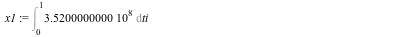(4)

and also this one:

x2:=sin(2*Pi*x1);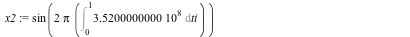(5)

I can then evaluate (4) and take sine(2Pi * the evaluation of (4)):

value(x1);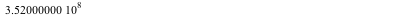(6)

sin(2*Pi*(6));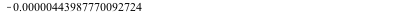(7)

Hmm... let's evaluate x2, which should be the same, right

value(x2);
0.00000556012229902952                                                              (8)

Oddly enough, it is not. Now the reason they are not 0 is due to round-off error (running the same sheet with Digits := 40 confirms that); but at the same time, (6) is in fact exact. More oddly, if I wrap the input leading to (7) in evalf() then it outputs 0., i.e. exact and correct. I suspect the problem must lie in the different treatments of Pi in the three cases.

I am not ready to call this behaviour a bug since I can see that different ways of evaluating what is essentially the same expression leads to a diffferent round-off. What strikes me is the relatively large errors in this case. The sheet was run with Digits being 15 (my default set in my .mapleinint), I initially expected somewhat more accuracy in the sine function than a mere 6 digits or so. On second thought, however, what is going on seems to be that the evaluation of the integral must be numerical and the large no. of cycles limits the accuracy; if one replaces 3.52E8 (a float) with 352E6 (an exact number) then (7) becomes 0 (exact) while (8) remains at the above value. Why

evalf(sin(2*Pi*(6)))

yields an exact value I do not quite understand.

So, caveat computor once again. This example, while it may look contrived, actually arose from a real-world case I was dealing with (the 352E6 is a frequency in Hz, in my actual application it can vary in time therefore the integration to get the no. of cycles in a given time interval). One annoyance here is that the "right" way to do this is not obvious, at least not to me.

M.D.

integration_test.mw

## Lattice Package Updated...

Maple

The Lattice package to investigate particle accelerator magnet lattices (original post) has been updated to V1.1. This is a significant update, addressing a number of inaccuracies and bugs of V1.0 as well as introducing new elements: Octupole, Fringe effect in dipoles, a MatchedSection allowing to insert a piece of beamline when the details are irrelevant, and a few experimental elements like WireQuad. New functions include the 6th synchrotron-radiation integral I6x, momentum compaction alphap and TaylorMap, which allows to compute the Taylor expansion of  the non-linear map to any degree.

The code and documentation are available in the Application Center.

U. Wienands, aka Mac Dude

## Lattice: A package to model accelerator ...

Maple

Magnet lattices for particle accelerators (the sequence of focusing and bending magnets and drift sections making up a beam line) are often designed numerically using computer codes like MAD that model each beam-line element using either a matrix description or numeric integration, or some other algorithm. The Lattice package for Maple—recently published in Maplesoft's Application Center—allows modelling such beam lines using the full algebraic power of Maple. In this way, analytic solutions to beam-optics problems can be found in order to establish feasibility of certain solutions or study parameter dependencies. Beam lines are constructed in an intuitive way from standard beam-optical elements (drifts, bends, quadrupoles etc.) using Maple's object-oriented features, in particular Records which represent individual elements or whole beam lines. These Records hold properties like the first-order transport matrices, element length and some other properties plus, for beam lines, the elements the line is composed of. Operations like finding matched Twiss (beam-envelope) functions and dispersion are implemented and the results can be plotted. The Lattice package knows about beam matrices and can track such matrices as well as particles in a beam. The tracking function (map) is implemented separately from the first-order matrices thus allowing nonlinear or even scattering simulations; at present sextupole elements, compensating-wire elements and scattering-foil elements take advantage of this feature. Standard textbook problems are programmed and solved easily, and more complicated ones are readily solved as well within the limits set by Maple's capabilities, memory and computing time. Many investigations are possible by using standard Maple operations on the relevant properties of the beam lines defined. An interesting possibility is, e.g., using Maple's mtaylor command to truncate the transfer map of a beam line to a desired order, making it a more manageable function.

The package can output a beam-line description in MAD8 format for further refinement of the solution, cross-checking the results and possibly more detailed tracking.

Developed initially for my own use I have found the package useful for a number of accelerator design problems, teaching at the US Particle Accelerator School as well as in modelling beam lines for experiments I have been involved in. Presently at Version 1.0 the package still has limits; esp. the higher-order descriptions are not yet as complete as desirable. Yet already many practical design problems can be tackled in great detail. The package is backwards compatible to Maple 15. It can be found in the Application center together with help database files (old and new style) and a Users Guide.

An example showing the flavor of working with the package is attached (FODO_example.mw). It analyzes a FODO cell, the basic cell used in many ring accelerators.

Uli Wienands, aka Mac Dude

## Random numbers...

Maple

Erik Postma from Maplesoft has recently posted a Maple application going through some of the ways how to produce random numbers in Maple: http://www.maplesoft.com/applications/view.aspx?SID=153662&P=TC-4444

Doing a fair bit of work involving random numbers (i.e. simulations) myself, I was naturally drawn to it. The document is a nice extension of the relevant help pages, making practical use of some of the procs in RandomTools easier and clearer.

One particular issue I recently ran into, however, is not covered. I needed a random generator for a particular custom pdf (not one of the already recognized pdfs) that happens to be 3-dimensional. And I needed to do this >twice<, with the second generation using the result of the first one as a parameter. the problem is not unlike the last example in the help page for Statistics:-Sample.

Here is what I did:

The pdf looks graphically like this (and note that in this plot it is not normalized):

plot3d(Triangle63:-Trianglef(CrystalAngle,DeflectionAngle),\
CrystalAngle=-0.8..0.2,DeflectionAngle=-0.6..0.6,projection=0.7);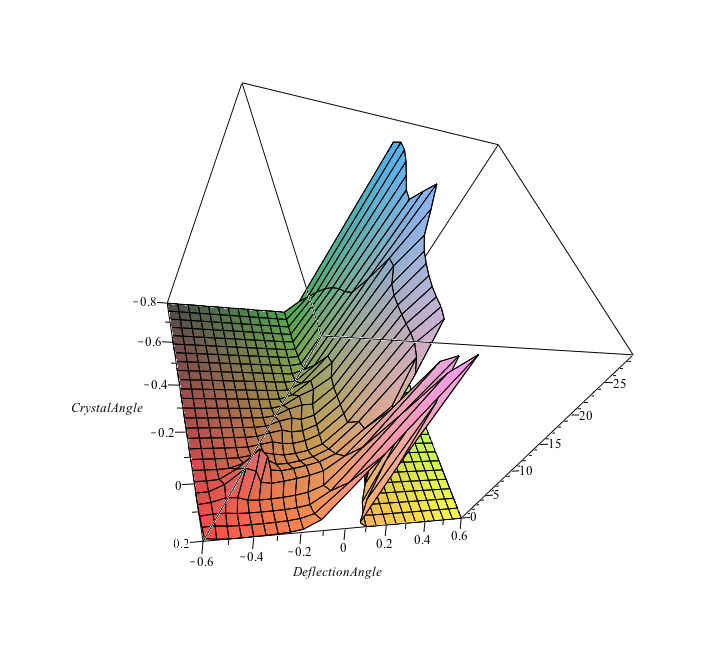The first set of random numbers is generated by setting CrystalAngle to a fixed number (-0.1 in this case), normalizing the pdf to the integral over DeflectionAngle and then generating the random numbers. Note that evaluation of the integral is relatively time-consuming (seconds).

trianglepdf:=(CrystalAngle,DeflectionAngle) ->\
Triangle63:-Trianglef(CrystalAngle,DeflectionAngle)/\
'evalf(Int(Triangle63:-Trianglef(CrystalAngle,Da),Da=-0.6..0.6,method = _d01akc))':
triangle0pdf:=(t) -> trianglepdf(-0.1,t):
Cr1:=Distribution(PDF=triangle0pdf);
Y:=RandomVariable(Cr1);

samples:=Sample(Y,[1..600],method=[envelope,range=-0.6..0.6]);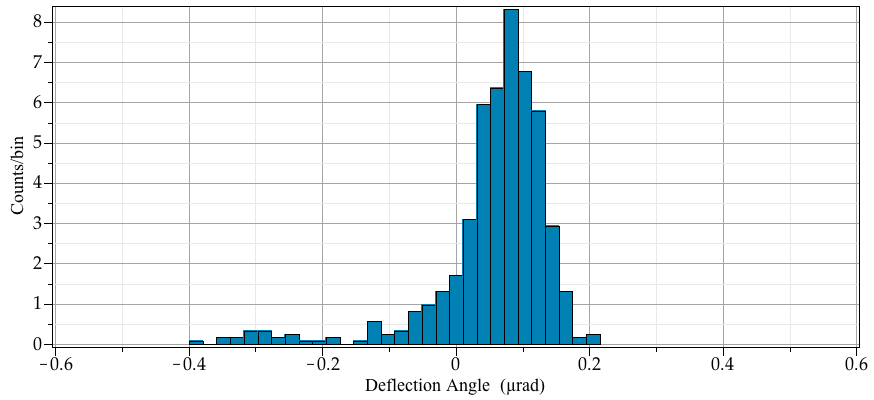This looks as it should; and the random number generation is reasonably fast once the initial setup has been done (i.e. the time used scales only relatively weakly with the count of random numbers generated.

The issue now is the second stage, where for each new random number, the number generated above is a new input parameter for CrystalAngle. So I can no longer define the pdf once since the pdf is different for each number generated.

The pedestrian way to do this is like the following:

samples2:=Vector(1..numelems(samples),datatype=float):
CrystalOffset:=0.3;

for ii from 1 to numelems(samples) do
triangleiipdf:=(t) -> (trianglepdf(samples[ii]-~CrystalOffset,t)); # subtract (VR peak -> 0)
Crii:=Distribution(PDF=triangleiipdf);
samples2[ii]:=evalf(Sample(RandomVariable(Crii),1,method=[envelope,range=-0.6..0.6])+CrystalOffset);
end do:

This works, but only sort-of. First, it is very slow, since the whole sertup happens for each number generated (Sample()). Secondly, it tends to hang at a certain number of steps through the loop. The value of ii where it hangs is arbitrary and changes with the other parameters and CrystalOffset. It is however consistent for identical runs.

The last example in help for Statistics:-Sample would indicate that one needs to setup the pdf as a function of t as well as the parameter, call Distribution() only once and then assign the value to the parameter and call Sample() to get the random number(s) drawn from the pdf with the parameter being set to the wanted value. While this works in the example which uses a built-in pdf, I find that I cannot make it work with my pdf. Either the parameter gets ignored (i.e. stuck at the first value) or the thing runs just as slowly as my procedure above.

Ultimately I programmed my own random generator for an arbitrary pdf which, while not as efficient as Maple's built-in generator, does produce the expected set of random numbers for the 2nd path in a reasonable time. It is a simple-minded envelope-rejection method, that works fo reasonably well-behaved pdfs:

Rarbit:=proc(pdf,xmin,xmax,pdfmax)
local dx:=xmax-xmin;
local x1,x2;
x1:=RandomTools[MersenneTwister]:-GenerateFloat64()*dx+xmin; # pick location on x axis
x2:=RandomTools[MersenneTwister]:-GenerateFloat64()*pdfmax; # y axis, probability of returning x1

while (x2>evalf(pdf(x1))) do # if above pdf, reject
x1:=RandomTools[MersenneTwister]:-GenerateFloat64()*dx+xmin; # new x-axis value
x2:=RandomTools[MersenneTwister]:-GenerateFloat64()*pdfmax; # check value
end do; # eventually we'll succeed and return one.

x1;
end proc;

Using this routine I get my second and final set of randome:

for ii from 1 to numelems(samples) do
newpdf:=(DefAng) -> Triangle63:-Trianglef(samples[ii]-CrystalOffset,DefAng);
samples2[ii]:=Random:-Rarbit(newpdf,-0.6,0.6,28);
DocumentTools:-SetProperty(ProgressBar,'value',ii,refresh);
end do:
CrystalOffset := 0.3
Histogram(samples2+~samples,view=[-0.6..0.6,default]);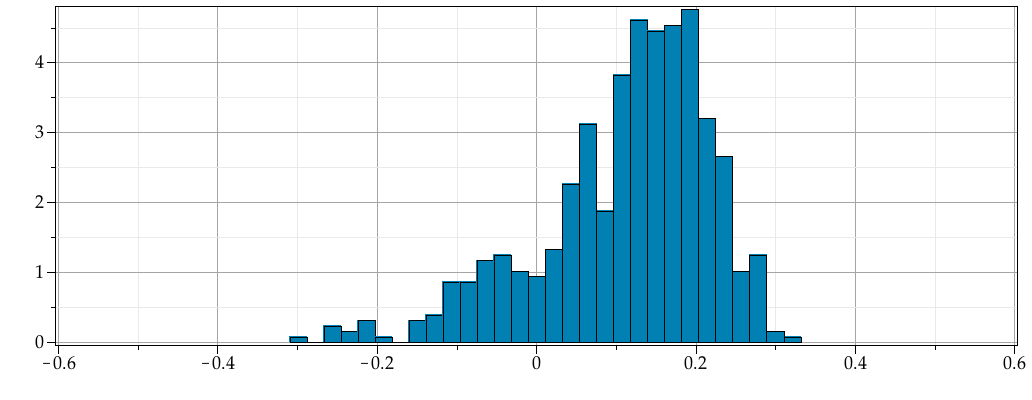I would be interested in Erik's comment on this.

Mac Dude

## MaplePrimes broken...

As others have noticed, after the update MaplePrimes is broken. I was not able to reply to the answers I got to my recent question about bookmarking. If someone listens: I am using Firefox 19.0 on Mac OS X 10.6.8.

I don't know how others feel, but if this is not addressed I am not sure how much more I will post here. MaplePrimes has been a vital forum for support for me and I have profited from all your help. If there is no more replying and discussion, this place has...

 Page 1 of 1
﻿